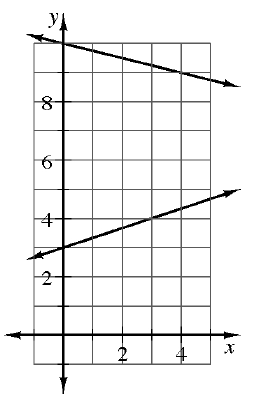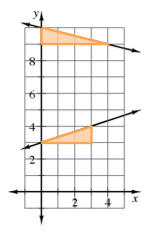### Home > CCAA8 > Chapter 10 Unit 11 > Lesson CCA: 10.3.1 > Problem10-108

10-108.Examine the two lines graphed at right. Will these two lines intersect? Find the equation of each line and test your prediction.

Find the slopes of each line by making slope triangles for each.
Look at the graph to determine the $y$-intercept for each line.Use the Equal Values Method to solve the system.
Is there a solution?

Use the eTool below to test your prediction.
Click on the link at right for the full eTool version: 10-108 HW eTool